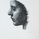27096 뷰
//Author - Rajandran R
//www.marketcalls.in
study("Supertrend V1.0 - Buy or Sell Signal", overlay = true)

Factor=input(3, minval=1,maxval = 100)
Pd=input(7, minval=1,maxval = 100)

Up=hl2-(Factor*atr( Pd ))
Dn=hl2+(Factor*atr( Pd ))

TrendUp=close>TrendUp? max(Up,TrendUp) : Up
TrendDown=close<TrendDown? min(Dn,TrendDown) : Dn

Trend = close > TrendDown ? 1: close< TrendUp? -1: nz (Trend,1)
Tsl = Trend==1? TrendUp: TrendDown

linecolor = Trend == 1 ? green : red

plot( Tsl , color = linecolor , style = line , linewidth = 2,title = "SuperTrend")

plotshape(cross(close, Tsl ) and close>Tsl , "Up Arrow", shape.triangleup,location.belowbar,green,0,0)
plotshape(cross( Tsl ,close) and close<Tsl , "Down Arrow", shape.triangledown , location.abovebar, red,0,0)
//plot(Trend==1 and Trend==-1,color = linecolor, style = circles, linewidth = 3,title="Trend")

plotarrow(Trend == 1 and Trend == -1 ? Trend : na, title="Up Entry Arrow", colorup=lime, maxheight=60, minheight=50, transp=0)
plotarrow(Trend == -1 and Trend == 1 ? Trend : na, title="Down Entry Arrow", colordown=red, maxheight=60, minheight=50, transp=0)

## 코멘트jordanovitch
@jordanovitch, can you share me how to set up the alarm,ths.
응답Thank you for sharing this. Can you please explain if it is possible to create an alert on "Up Entry Arrow"?
응답